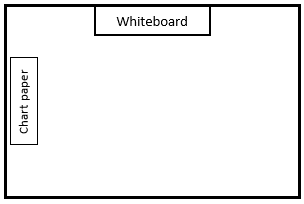Match Fishtank is now Fishtank Learning!

# Multiplication and Division, Part 1

## Objective

Demonstrate the commutativity of multiplication.

## Common Core Standards

### Core Standards

?

• 3.OA.B.5 — Apply properties of operations as strategies to multiply and divide. Students need not use formal terms for these properties. Example: Knowing that 8 × 5 = 40 and 8 × 2 = 16, one can find 8 × 7 as 8 × (5 + 2) = (8 × 5) + (8 × 2) = 40 + 16 = 56. (Distributive property.) Example: If 6 × 4 = 24 is known, then 4 × 6 = 24 is also known (Commutative property of multiplication.) 3 × 5 × 2 can be found by 3 × 5 = 15, then 15 × 2 = 30, or by 5 × 2 = 10, then 3 × 10 = 30. (Associative property of multiplication.)

?

• 2.NBT.A.2

• 2.OA.C.3

• 2.OA.C.4

## Criteria for Success

?

1. Understand that a column is a vertical group in an array.
2. Know that when multiplying numbers, the order in which factors are multiplied doesn’t matter (MP.7).
3. Understand that the above property is called the commutative property
4. Demonstrate and explain the commutativity of multiplication using models (MP.3).
5. Use the commutative property to solve multiplication factors where students are able to solve by switching factors (e.g., 5 x 8 becomes 8 x 5 so that students can count by fives, the size of each group).

## Tips for Teachers

?

Students need not use formal terms for the properties of operations, including the terms “commutative” or “commutative property.” However, exposure to the term is helpful so that students can develop and use a common language and thus is introduced in this lesson.

#### Remote Learning Guidance

If you need to adapt or shorten this lesson for remote learning, we suggest prioritizing Anchor Task 2 (benefits from worked example). Find more guidance on adapting our math curriculum for remote learning here.

#### Fishtank Plus

• Problem Set
• Student Handout Editor
• Vocabulary Package

?

### Problem 1

1. Mr. Barron is working with a small group of students. He sets up their seats facing the whiteboard into two rows with three chairs in each row.

a. Draw the seats in Mr. Barron’s room below, using squares to represent the seats.b. Write a multiplication equation that can be used to find the total number of seats Mr. Barron has in the room.

c. Determine the total number of seats in Mr. Barron’s room.

2. Sometimes Mr. Barron wants to use chart paper on the side of his room, which you can see in the diagram above. Imagine students facing Mr. Barron at the chart paper.

a. Write a multiplication equation that can be used to find the total number of seats Mr. Barron has in the room.

b. Determine the total number of seats in Mr. Barron’s room.

3. What do you notice about #1 and #2? What do you wonder?

### Problem 2

$4 \times 5 = 5\times 4$

### Problem 3

Solve.

a.   $2\times 8 =$ _____

b.   _____ $= 10\times 7$

## Problem Set & Homework

#### Discussion of Problem Set

• We don’t know our 3 or our 6 count-bys yet. How were you able to solve #1?
• How did the commutative property help you to solve the equations in #7?
• Are there other operations besides multiplication where you can switch the order of the numbers without having an effect on the solution? Which ones?

?

 $2\times 5 = 5\times 2$

Do you agree or disagree with the statement in the box? Draw a picture to explain your answer.

#### References

EngageNY Mathematics Grade 3 Mathematics > Module 1 > Topic C > Lesson 7Exit Ticket

Grade 3 Mathematics > Module 1 > Topic C > Lesson 7 of the New York State Common Core Mathematics Curriculum from EngageNY and Great Minds. © 2015 Great Minds. Licensed by EngageNY of the New York State Education Department under the CC BY-NC-SA 3.0 US license. Accessed Dec. 2, 2016, 5:15 p.m..

Modified by Fishtank Learning, Inc.

### Mastery Response

?